Courses

# Small Oscillations and Normal Modes - Lagrangian and Hamiltonian Equations, Classical Mechanics, CSI Physics Notes | EduRev

## Physics for IIT JAM, UGC - NET, CSIR NET

Created by: Akhilesh Thakur

## Physics : Small Oscillations and Normal Modes - Lagrangian and Hamiltonian Equations, Classical Mechanics, CSI Physics Notes | EduRev

The document Small Oscillations and Normal Modes - Lagrangian and Hamiltonian Equations, Classical Mechanics, CSI Physics Notes | EduRev is a part of the Physics Course Physics for IIT JAM, UGC - NET, CSIR NET.
All you need of Physics at this link: Physics

Small Oscillations: One degree of freedom.

Let us begin by considering the motion of a one-dimensional system near a critical point of the potential energy. While only the simplest systems can be reduced to one degree of freedom, this case is still particularly important because { as we shall soon see { multi-dimensional systems (in the vicinity of stable equilibrium) can be reduced to multiple copies of the one dimensional case.

We assume the Lagrangian is of the form: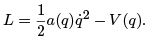Note in particular that we assume (for now) that the system is closed, i.e., energy is conserved. Let q0 be a critical point of the potential, so that V'(q0) = 0. Let us approximate the motion by assuming that x := q - q0 is "small". We expand the Lagrangian in a Taylor series in x, keeping only the rst non-trivial terms (exercise):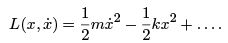Here we have set m = a(q0) and k = V"(q0). Of course, the kinetic energy must be positive, so that m > 0. Note that we also have dropped the irrelevant constant V (q0). If desired we can adjust the zero point of potential energy so that this constant is zero.
The EL equations are (in the domain of validity of the approximation)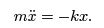This equation is easily integrated: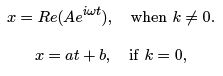where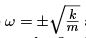and A is a complex constant encoding the two real integration constants, which can be xed by initial conditions. If k > 0, then q0 is a point of stable equilibrium, and we get harmonic motion. In particular, if x is small initially and the initial velocity is suciently small, then x(t) remains small (exercise), so that our approximation is self-consistent. On the other hand, if k ≤ 0, then the motion of the particle need not maintain our approximation of small x, our approximation is not self-consistent and must be abandoned after a very short time. Of course, the case k < 0 corresponds to unstable equilibrium, for which a small perturbation leads to a rapid motion away from equilibrium.
If k = 0, then the critical point q0 is not a maximum or minimum but is a saddle point ("neutral equilibrium"), our approximation again becomes invalid, although the time scale for this is larger than the case of unstable equilibrium.

Thus in a neighborhood of a point of stable equilibrium it is consistent to make the harmonic approximation to the potential and kinetic energies. In the harmonic approximation the motion of the system is mathematically the same as that of a simple harmonic oscillator.

A very familiar example of all of this is the planar pendulum of mass m and length l for which q = θ is the deffection from a vertical position. We have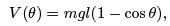and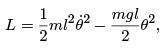The Lagrangian in the harmonic approximation near equilibrium at θ = 0 is (exercise)

so that the harmonic motion has \mass" ml2 and angular frequency"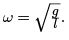(Exercise: what happens to motion near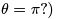Example

Consider a mass m which is constrained to move on a straight line. The mass is bound to a xed point by harmonic force with potential energy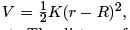where K is a constant, r is the distance of the particle to the xed point. The distance from the point to the line is l > R. A mechanical model of this system is a mass sliding on a straight track, the mass being connected to a xed point by a spring. Our goal is to nd the stable equilibrium position(s) and compute the frequency of small oscillations about the equilibrium. Evidently,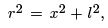where x is the position of the particle along the given line, with x = 0 the location at distance l from the center of force. Using x as the generalized coordinate, the Lagrangian for this system is (exercise)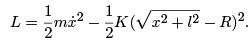You can check that x = 0 is the only point of stable equilibrium. (As an exercise you can check that the point x = 0 is a point of unstable equilibrium if we assume R ≥ l.) You can expand the potential to second order to nd that (exercise)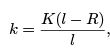so that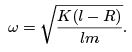Note that K (l R) is the force needed to move a particle from r = R to the point r = l (exercise) in the presence of the potential V .

Example

We return to our example of a plane pendulum of length l with horizontally moving point of support. The mass m1 (the point of support) has position x, and the angular deffection of the pendulum (mass m2) is denoted by φ. The kinetic energy is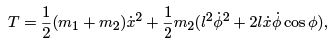and the potential energy is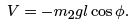We have already seen that we can use conservation of the momentum conjugate to x to effect a Lagrangian reduction which eliminates the x degree of freedom. In detail, the conservation law we need is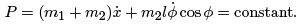This conservation law is just the conservation of the x component of the center of mass of the system (exercise).

Let us compute the frequency of small oscillations of the pendulum in the reference frame in which P = 0, so that (exercise)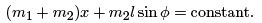In this reference frame, which is the rest frame of the x component of the center of mass, the reduced kinetic energy is (exercise)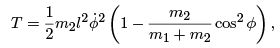and the reduced potential energy is (exercise)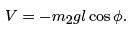Clearly φ = 0 is an equilibrium point (exercise). Expanding in powers of φ we get, in the harmonic approximation,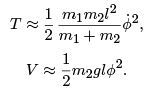It is now straightforward to see that the frequency of small oscillations is (exercise)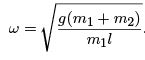Of course, in a generic inertial reference frame the motion of the system in the harmonic approximation is small oscillations at the above frequency superimposed with a uniform translation of the center of mass along the x direction.

As a sanity check, let us consider the limit in which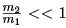, i.e., the mass at the point of support is becoming large. Physically, we expect that the point of support moves with a uniform translation along x. In the rest frame of the point of support (which is now the approximate center of mass) we have a traditional plane pendulum problem. In this limit we get (exercise)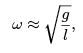as expected.

Oscillations of systems with more than one degree of freedom.

So far we have studied (small) oscillations of systems with a single degree of freedom. Systems with more degrees of freedom can exhibit much more intricate behavior in the vicinity of stable equilibrium. However, this more intricate behavior can always be viewed as the superposition of harmonic motions of decoupled degrees of freedom - the normal modes of vibration. We shall rst spend a little time developing the general theory, and then we shall spend some time on examples.

Suppose we have a system with n generalized coordinates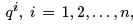and a Lagrangian of the form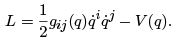Recall that we use the summation convention, there is a double sum in the rst term of L. The "metric" gij (q) is a symmetric array which may depend upon the con guration coordinates. There is no loss of generality by assuming the metric to be symmetric,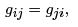since only the symmetric combination appears in the sum over i and j (exercise). Usually, the metric is diagonal (e.g., in spherical polar coordinates), but we have seen examples where the metric is off diagonal (e.g., pendulum with moving point of support).
Critical points of V , i.e., points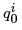such that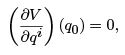define equilibrium configurations of the system (exercise). We will sometimes suppose that the equilibrium is stable, i.e.,is a (local) minimum of V . But you should always be thinking about the other types of equilibrium as we go.
Let us approximate the motion in the neighborhood of a point of equilibrium by de ning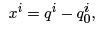and expanding the Lagrangian in a Taylor series about x= 0. To the rst non-trivial order we get (exercise)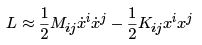where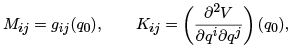and we have dropped an irrelevant additive constant V (q0), i.e., we have adjusted the zero of potential energy to be at. We have that Mij = Mji and we assume that the potential energy is sufficiently smooth so that the matrix of second partial derivatives is symmetric at the critical point q0 :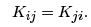The (approximate) EL equations are (exercise)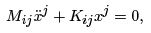which are coupled system of n homogeneous, linear ODEs with constant coefficients. Defining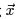as a column vector with entries xi, and viewing Mij and Kij as (symmetric) matrices M and K , we can write the EL equations in the matrix form (exercise):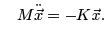Let us note that ifis a point of stable equilibrium then the symmetric matrix K is positive de nite, that is, it can have only positive eigenvalues.* This is because a negative or zero eigenvalue will correspond to displacements xi which either lower or do not change the potential energy in an arbitrarily small neighborhood of the equilibrium point (exercise), which contradicts our assumption of stable equilibrium. Conversely, since every symmetric matrix can be diagonalized, if the eigenvalues are all positive defi nite then the pointis a minimum. Put differently,is a point of stable equilibrium if and only if the quadratic form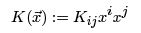is positive de finite, which means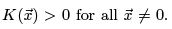. Physically this means that any displacement xi from equilibrium will increase the potential energy. All this discussion is just restating standard results from multivariable calculus.

Likewise, positivity of the kinetic energy implies that in any physical application the symmetric matrix M should be positive de nite. This means the quadratic form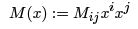is positive de nite, i.e.,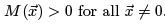We assume this in what follows.
Here's our strategy for analyzing the (approximate) EL equations. We can write the EL equations as (exercise)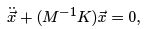where M -1 exists because M is positive definite (exercise). Suppose we can nd an eigenvector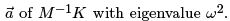. (For now, we allow ω to be complex.) Then it is easy to see that we will get a solution the form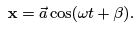Thus, provided ω2 > 0, i.e., ω is real and non-vanishing, some combination of the displacements of the system (determined by the eigenvector) is behaving as a harmonic oscillator with frequency ω. If we can nd enough eigenvectors, we will be able to build the general solution to the EL equations by superposition. Because the general solution should involve 2n integration constants — corresponding to initial positions and initial velocities, we will need n independent solutions of the above form (exercise), i.e., n linearly independent eigenvectors, to get the general solution in this manner.

Our goal, then, is to determine the eigenvalues ω2 and the eigenvectors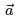. We will write our eigenvalue equation in the equivalent form (exercise):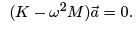Thusis an eigenvector of the matrix (K - ω2M ) with eigenvalue zero. You will recall the basic result from linear algebra that the square matrix K - ω2M has an eigenvector with eigenvalue zero if and only if (exercise)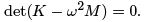This is the characteristic equation for ω, it is a polynomial equation for ω of order 2n. Thus ω, called the characteristic frequency, arises as a root of a polynomial of order 2n.
In general there are 2n roots of the characteristic equation. But notice that if ω is a solution, then so is -ω since they both yield the same ω2. Since it is ω2 which determines the eigenvector, we see that changing the sign of ω does not give a new solution (linearly independent eigenvector). Thus, without loss of generality, we can assume that ω > 0. Thus we get n characteristic frequencies. At this point, as far as we know, these frequencies may be non-vanishing and real, or zero, or imaginary. The real solutions correspond to stable directions in con guration space. The imaginary solutions correspond to directions in con guration space relative to which the equilibrium is unstable. The vanishing frequencies correspond to directions in con guration space relative to which we have neutral equilibrium. Corresponding to each of these roots is an eigenvectorand hence a solution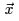to the EL equations.

It is not hard to see that if the potential energy quadratic form is positive de nite then ω2 > 0, so that there are n real, positive characteristic frequencies. To see this, simply note that for any displacementsolving the equations of motion we have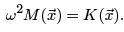Because both quadratic forms are positive de nite,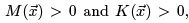, it follows that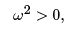as desired.
Physically speaking, the existence of n positive roots for ω stems from our assumption that q0 is a point of stable equilibrium, which mathematically means K () is positive definite. If K () could be negative or zero, allowing for complex or vanishing frequencies, this would lead to exponential or linear (rather than oscillatory) solutions. Henceforth we assume we have stable equilibrium and just focus on the sinusoidal solutions. You should have no problem adapting our discussion to the other cases (and we will have an example of neutral equilibrium in a little while)

Once we have a characteristic frequency ω, we can reconstruct the corresponding ai by solving the equation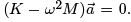The solution is guaranteed to exist (because ω solves the characteristic equation). In this way we have, in fact, found an eigenvector () of M -1K with eigenvalue ω2 (exercise).

It is, in general, possible to find n roots of the characteristic equation along with n orthogonal - and hence linearly independent - eigenvectors (more on this shortly). Each of the (real) solutions obtained through this procedure evolves in time harmonically at the characteristic frequency, these solutions are called normal modes. Let us denote the characteristic frequencies by ωα and the corresponding normalized eigenvectors by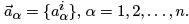The general solution to the EL equations is then a superposition of the normal modes: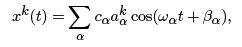where cα and βα are constants (of integration) determined by initial conditions.

Normal Modes - The Recipe

Let us summarize the construction of the normal modes of vibration described by the Lagrangian, which approximates the dynamics of a system near equilibrium: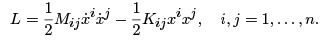First we solve the characteristic equation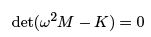for the characteristic frequencies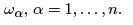We then solve the linear equations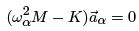for the corresponding vectors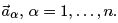The normal modes of vibration are*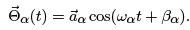The general motion of the system in the vicinity of stable equilibrium (in the harmonic approximation) is a superposition of the normal modes. The superposition goes over the amplitudes and phases of each oscillator. These coefficients are determined by initial conditions. We have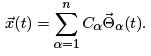An Elementary Example

As a very simple example of nding normal modes and characteristic frequencies, let us consider a system described by the Lagrangian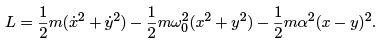This system can be viewed as two identical one-dimensional harmonic oscillators (natural frequency ω0 ) with a coupling by a harmonic force (natural frequency α) (exercise).
The matrices M and K are given by (good exerciseω)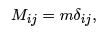and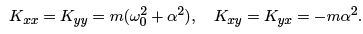We now have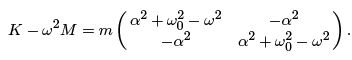The characteristic equation is (exercise)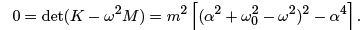The characteristic frequencies are then given by (exercise)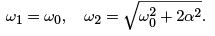The corresponding normalized normal modes are determined by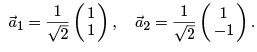We have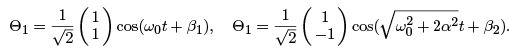Evidently, the oscillation at frequency ω0 corresponds to the two masses moving exactly in phase, so the coupling does not come into play, while the oscillation at frequency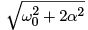has the two masses moving exactly out of phase.
The general motion of the system is a superposition of these two normal modes. We can write the general motion of the system in vector form as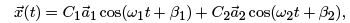or, more explicitly,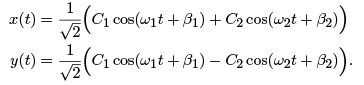Here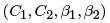are real constants which are determined by initial conditions. Despite the fact that the motion is very regular, i.e., it is the superposition of harmonic oscillations, the appearance of the motion can be quite complicated.

Example: Double Pendulum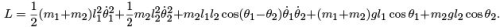Recall that mhas a xed point of support, while m2 is supported at the location of m1.
Stable equilibrium occurs at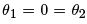We expand in Taylor series about equilibrium to get the approximate Lagrangian: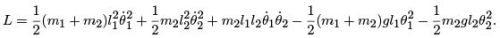Note that the coupling between degrees of freedom occurs through the kinetic terms. The characteristic equation is (exercise)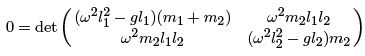The roots are (exercise)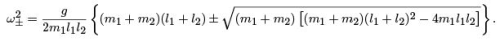To carry on, let us consider a special case. Suppose the two pendula are identical: m= m2 = m, l1 = l2 = l. Then the characteristic frequencies become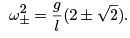Note that all dependence upon the mass drops out. In this case the normal modes are determined by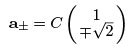The normal mode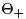has both masses having velocities out of phase, the normal mode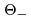has the velocities in phase.

Example: Linear triatomic molecule

As another application of our theory let us consider the motion near equilibrium of a linear triatomic molecule. By this we mean that we have two atoms of mass m located symmetrically on either side of an atom of mass M . Let all three atoms lie on a line.
For simplicity we only consider longitudinal motion, i.e., motion along the extent of the molecule. We assume that, near equilibrium, the restoring force on the atoms is kx, where x is the displacement of an atom from equilibrium. In detail, let x1 and x2 denote the longitudinal displacements of each m from equilibrium, and let X denote the displacement of M from equilibrium. The potential energy is approximated by (exercise)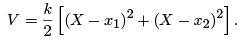The kinetic energy is simply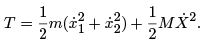Ordering the coordinates on the con guration space as (x1, x2, X ), the matrices of interest are (exercise)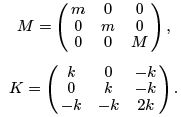The characteristic equation is (exercise)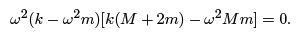The solutions are (exercise)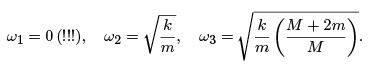The appearance of a zero frequency mode is, at rst, a little disconcerting, but simply respects the possible motion in which all three masses move with a uniform translation (see below). The other two modes represent oscillations near equilibrium. To see all this, we need to compute the normal modes. We get (exercise)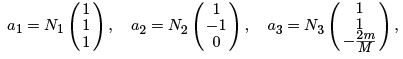where the N 's are normalization constants. The motion of mode 1 is rigid motion at constant velocity. To see this we simply note that

x1 = x2 = X = at + b

solves the equations of motion and with zero frequency (exercise). Note that a spatial translation of the system is a symmetry of the Lagrangian, so the total (center of mass) momentum is conserved. If desired, we can work in the rest frame of the center of mass; this reduction eliminates the center of mass single degree of freedom and leaves two oscillatory degrees of freedom. The motion of mode 2 has the central atom at rest and the two endpoint atoms oscillating 180 degrees out of phase with the same amplitude. The third mode has the endpoint atoms in phase, with the same amplitude while the center atom moves 180 degrees out of phase with them and with a di
erent amplitude. These latter two modes keep the center of mass at rest.

Finally, let us briefly and qualitatively consider the general, non-longitudinal motion of the molecule. Of course, if we allow for vibrations perpendicular to the line de ned of the molecule we have more degrees of freedom (namely, 9) to consider. While the explicit computations are more lengthy, nothing conceptually new arises. There will be a number of zero frequency modes corresponding to rigid motions (translations and rotations) of the molecule. The remaining modes will be truly vibrational. Of the 9 degrees of freedom and the 9 corresponding modes, 5 will be of the zero-frequency type (exercise). Naively, there are 3 translations and 3 rotations, but the linear nature of the molecule, and its modeling via point masses, means that rotations about the molecular axis are not motions of the system. Thus there are only 5 zero frequency modes: 3 translations + 2 rotations. This leaves 4 vibrational modes. Two of these modes{the longitudinal modes{we have already studied. The other two modes are transverse to the axis of the molecule. If we think of this axis as the x-axis, then the transverse vibrations come from displacements in the y and z directions. Clearly there is nothing to physically distinguish y from z, so we expect that the frequencies for the two transverse normal modes will be degenerate. Indeed, there is nothing to pick out which orthogonal directions to the molecule should be y and z. The system exhibits a symmetry under rotations about the molecule axis. Thus the normal modes for transverse vibrations will be along any two perpendicular directions each of which is perpendicular to the molecule axis.

The rigid motions of the molecule, which give rise to the zero frequency modes, correspond to symmetries and conservation laws. Since the potential energy is changed only by a relative motion of the atoms, it clearly will exhibit a symmetry with respect to any transformation that leaves the relative position of the atoms unchanged, i.e., rotations and translations of the molecule as a whole. The kinetic energy is, of course, invariant under such transformations (exercise). Thus the Lagrangian is invariant under the rigid rotations and translations of the molecule. The corresponding conservation laws are 5 in number (3 translations and 2 rotations{exercise); they are the 3 components of the center of mass momentum (no external forces) and 2 components of angular momentum (no external torques).

Offer running on EduRev: Apply code STAYHOME200 to get INR 200 off on our premium plan EduRev Infinity!

159 docs

,

,

,

,

,

,

,

,

,

,

,

,

,

,

,

,

,

,

,

,

,

,

,

,

,

,

,

;